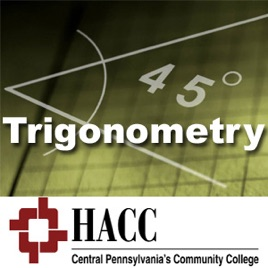32 episodes

Trigonometric functions, relationships, and graphs; identities and trigonometric equations; composite, multiple, and half-angle formulas; complex numbers; DeMoivre's theorem.# MATH 104: Trigonometry - iPod Video Harrisburg Area Community College

• Education

Trigonometric functions, relationships, and graphs; identities and trigonometric equations; composite, multiple, and half-angle formulas; complex numbers; DeMoivre's theorem.

## Customer Reviews

3.9 out of 5
47 Ratings

47 Ratings

ZOPikeCPL ,

### Great course - but missing too many Chapters

Thank you for providing this course online! I just wish you included more from Chapter 4. Such as graphing the other trigonometric functions.

sooohaib92 ,